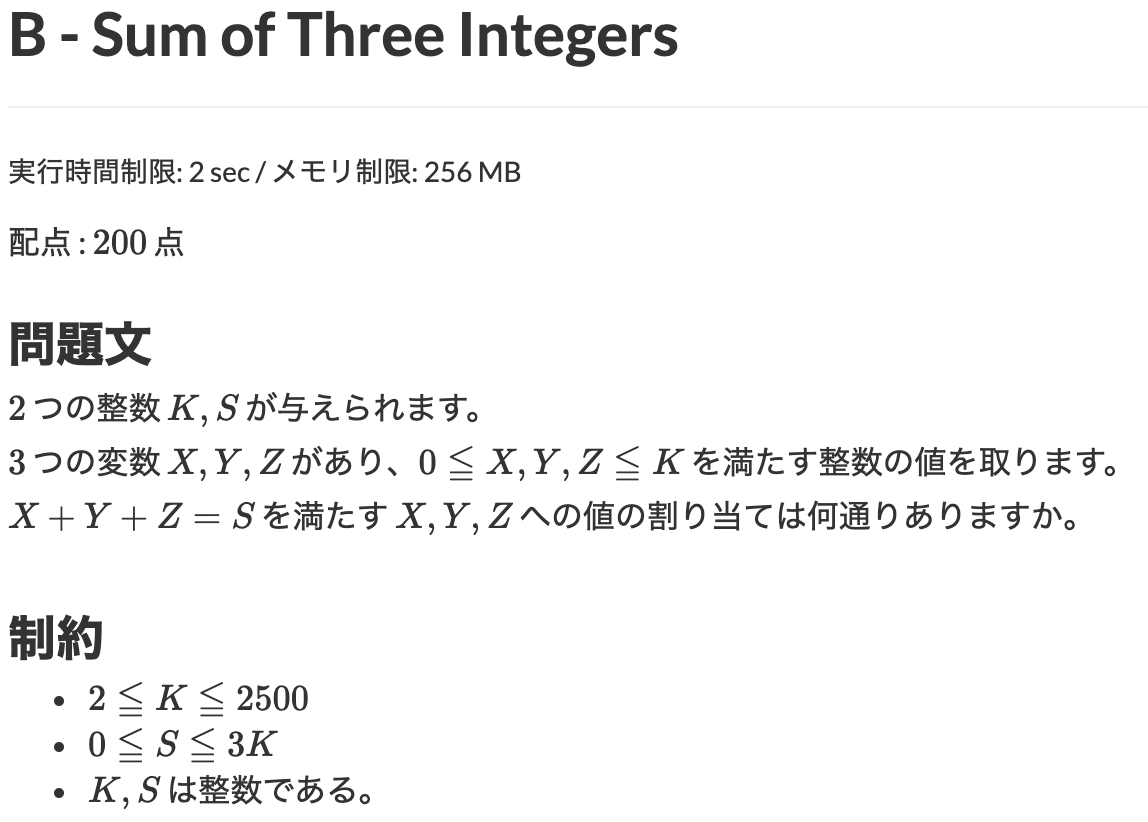# ABC051 B - Sum of Three Integers

atcoder# # Problem

Given integers K and S, report the number of combinations of integers X, Y, Z which satisfy $0 \leq X, Y, Z \leq K$ and $X + Y + Z = S$.

# # Time complexity

$O(K^2)$

# # Solution

#define MAX_K 2501
#define MAX_S 3 * MAX_K

Int K, S;

void input() {
cin >> K >> S;
}

void solve() {
Int count = 0;
loop(x,0,K+1) {
loop(y,0,K+1) {
Int z = S - x - y;
if (0 <= z && z <= K) count++;
}
}
cout << count << endl;
}

int main(void) {
input();
solve();
}Remote freelancer. A web and mobile application enginner.
Traveling around the world based on East Asia.
I'm looking forward to your job offers from all over the world!

Offer jobs or contact me!